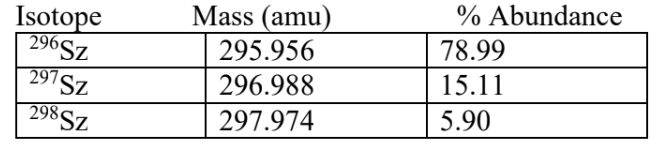# Problem: Pretend that a new element named Szulczewskium (Sz) has been discovered. It is found to have three naturally occurring isotopes. Use the information below to calculate the atomic mass of Sz that would appear in the periodic table.a. 296.0 amub. 296.2 amuc. 296.4 amud. 297.0 amue. 297.5 amu

###### FREE Expert Solution

We’re being asked to calculate the  calculate the atomic mass of Szulczewskium (Sz) that would appear in the periodic table. We can use the following equation:

where atomic mass = average atomic mass of the element and f.a. = fractional abundance of the isotope. To get f.a., we simply need to divide the given percent abundance by 100.

81% (377 ratings)###### Problem Details

Pretend that a new element named Szulczewskium (Sz) has been discovered. It is found to have three naturally occurring isotopes. Use the information below to calculate the atomic mass of Sz that would appear in the periodic table.a. 296.0 amu
b. 296.2 amu
c. 296.4 amu
d. 297.0 amu
e. 297.5 amu